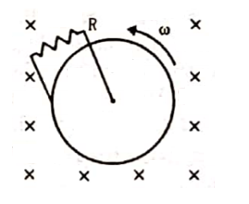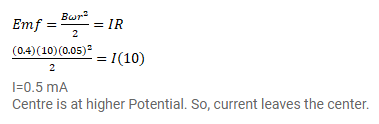# Figure shows a conducting disc rotating about its axis in a perpendicular magnetic field B.

Question:

Figure shows a conducting disc rotating about its axis in a perpendicular magnetic field $B$. A resistor of resistance $R$ is connected between the center and the rim. Calculate the current in the resistor. Does it enter the disc or leave it at the center? The radius of the disc is $5.0 \mathrm{~cm}$, angular speed $\omega=10 \mathrm{rad} / \mathrm{s}, \mathrm{B}=0.40 \mathrm{~T}$ and $\mathrm{R}=10 \Omega$.Solution: